Next: Example Up: Budan-Fourier method Previous: Mathematical background   Contents

Implementation

This procedure is used to determine the number of real roots in a given interval, up to an even number. The syntax of the procedure is:

INT Budan_Fourier_Interval(int Degree,VECTOR &Coeff,INTERVAL In)
INT Budan_Fourier_Interval(int Degree,INTEGER_VECTOR &Coeff,INTERVAL In)
with:
• Degree: degree of the polynomial
• Coeff: the Degree+1 coefficients of the polynomial in increasing degree, which may be REAL or INT
• In: the interval in which we are looking for the number of roots
If this procedure returns the integer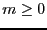, then the number of real roots in In is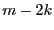with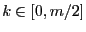. A negative returns code indicate a failure of the algorithm:
• -1: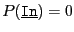• -2: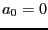, the polynomial may be factored
• -3: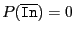This procedure may be used with polynomial whose coefficients are intervals. The syntax is:

INT Budan_Fourier_Interval(int Degree,INTERVAL_VECTOR &Coeff,INTERVAL In,int *Confidence)
where Confidence is a quality index for the result:
• 1: the result is exact, so if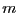is the return code of the algorithm the number of reals roots of the interval polynomial iswith•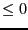: ifis the return code of the algorithm the number of reals roots of the interval polynomial is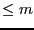Due to rounding errors incorrect results may be returned by the previous procedures. A safer procedure is:

INT Budan_Fourier_Safe_Interval(int Degree,VECTOR &Coeff,
INTERVAL In,INTERVAL &NbRoot);
The procedure returns 1 in case of success and an interval for the number of roots. If NbRoot=[a,b], then if a =b the number of roots is either a,a-2,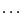and if a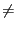b the number of roots is lower than b. If "safe" value of the coefficients have been pre-computed you may use:

INT Budan_Fourier_Fast_Safe_Interval(int Degree,INTERVAL_VECTOR &Coeff,
INTERVAL In,INTERVAL &NbRoot);
Another safe procedure is:

INT Budan_Fourier_Interval(int Degree,INTEGER_VECTOR &Coeff,int Inf,int Sup)
where the coefficients and the bounds are integers.Next: Example Up: Budan-Fourier method Previous: Mathematical background   Contents
Jean-Pierre Merlet 2012-12-20